•5星
40KB weixin_51194902 2021-01-08 12:19:19
• javascript数据结构和算法——哈希表 javascript 数据结构 算法 哈希表

javascript数据结构算法——哈希哈希函数：将一个字符串转化成对应的下标的封装程序。 哈希表：据关键码值(Key value)而直接进行访问的数据结构。 哈细化：一个关键字将其转化成对应的数字，再将这个数据映射到...

javascript数据结构和算法——哈希表

哈希函数：将一个字符串转化成对应的下标的封装程序。
哈希表：据关键码值(Key value)而直接进行访问的数据结构。
哈细化：一个关键字将其转化成对应的数字，再将这个数据映射到对应的内存空间的范围，而这一映射的过程就是哈希化。
应用场景：
①如果在一个公司中，我想要根据一个人名，来查找这个人的全部信息如果按照直接查找，效率太低。而使用哈希表，可以将人名转化成对应的下标，根据下标来查找，可以提高效率。
②、可以应用在字典的查找与搜索过程中。
步骤一：
先将对应的字符根据对应的编码转化成数字。
步骤二
因为步骤一得到的数字太大，所以可以进行哈希化，就是根据一个操作将其转化成给定范围的地址。
步骤三:
如果出现冲突的情况，可以有两种操作的：一：开放地址法，二：链地址法，接下来的操作我们会使用链地址法进行相关的操作。
链地址法“：上面的图就是链地址法的相关操作，将地址重复的数据全部在用指针指向一个数组。
代码操作
这里我们将哈希表封装成一个构造函数
需要的数据：①整个哈希表全部数据
②哈希表中限制长度
③哈希表中的元素数量
需要的方法：
①放入元素
②获取元素
③修改元素
④删除元素
⑤判断是否为空
⑥输出元素个数
⑦扩展空间

<script>
function hashTable() {
this.storage = [];
this.limit = 7;
this.count = 0;
hashTable.prototype.hashFunc = function (str, size) {
let hashCode = 0;
for (let i = 0; i < str.length; i++) {
hashCode = hashCode * 37 + str.charCodeAt(i);
}
let hash = hashCode % size;
return hash;
}
// 添加、更改哈希函数中的值
hashTable.prototype.push = function (key, value) {
// 先用哈希函数将其转化
let index = this.hashFunc(key, this.limit);
let bucket = this.storage[index];
if (bucket == null) {
bucket = [];
this.storage[index] = bucket;
}
for (let i = 0; i < bucket.length; i++) {
if (key == bucket[i]) {
bucket[i] = value;
return;
}
}
// 指向到这里就不存在，则直接插入
bucket.push([key, value]);
this.count++;
if (this.count >= this.limit * 0.75) {
this.limit = this.getPrime(this.limit * 2);
this.resize(this.limit);
}
}
// 获取操作
hashTable.prototype.get = function (key) {
let index = this.hashFunc(key, this.limit);
let bucket = this.storage[index];
if (bucket == null) {
return null;
}
for (let i = 0; i < bucket.length; i++) {
if (key == bucket[i]) {
return bucket[i];
}
}
// 执行到这里说明不存在该值
return null;
}
// 删除方法
hashTable.prototype.remove = function (key) {
let index = this.hashFunc(key, this.limit);
let bucket = this.storage[index];
if (bucket == null) {
return null;
}
for (let i = 0; i < bucket.length; i++) {
if (key == bucket[i]) {
this.count--;
if (this.limit <= 7 && this.count <= this.limit * 0.25) {
this.limit = this.getPrime(this.limit);
this.resize(this.limit);
}
return bucket[i];
}
}
return null;
}
// 判断是否哈希表是否为空
hashTable.prototype.isEmpty = function () {
return this.count == 0;
}
// 输出哈希表的长度
hashTable.prototype.size = function () {
return this.count;
}
// 判断是否为质数
hashTable.prototype.isPrime = function (num) {
let sqrt = Math.sqrt(num);
for (let i = 2; i <= sqrt; i++) {
if (num % i == 0) {
return false;
}
}
return true;
}
// 获取一个质数
hashTable.prototype.getPrime = function (num) {
while (!this.isPrime(num)) {
num++;
}
return num;
}
// 设置扩容，缩容操作
hashTable.prototype.resize = function (newList) {
// 重新定义变量
let oldStorage = this.storage;
this.storage = [];
this.count = 0;
this.limit = newList;
for (let i = 0; i < oldStorage.length; i++) {
let busket = oldStorage[i];
if (busket == null) {
continue;
}
for (let j = 0; j < busket.length; j++) {
var tuple = busket[j];
this.push(tuple,tuple);
}
}
}
}
let hs = new hashTable();
hs.push("dl", '201902091');
hs.push("xsy", "201902018");
console.log(hs);
hs.push("zcc", '201902091');
hs.push("bjr", "201902018");
hs.push("fzl", '201902091');
hs.push("hst", "201902018");
console.log(hs);
</script>

解析：上面是完整的代码，其中包含扩容和缩容的操作，原因是：在哈希表中，如果在多余的元素中，使用链地址法，则可以存放无数个元素，当元素的个数多的时候，查找时，消耗的时间以及其效率都会增多。所以当满足某一个条件时，执行扩容操作。当满足某一个条件时，进行缩容操作，因为当元素个数较少时，如果容积比较大，比较浪费内存空间。
并且在上面代码中，增加了查找质数的函数，因为当哈希表的空间范围是质数的时候，元素分布比较均匀。

展开全文weixin_47450807 2021-02-16 15:14:51
• 相似图像搜索的哈希算法思想及实现（差值哈希算法和均值哈希算法） 算法 python opencv hash 计算机视觉

图像相似度比较哈希算法: 什么是哈希（Hash）？ • 散列函数（或散列算法，又称哈希函数，英语：Hash Function）是一种从任何一种数据中创建小 的数字“指纹”的方法。散列函数把消息或数据压缩成摘要，使得数据量变...

图像相似度比较哈希算法:

什么是哈希（Hash）？

• 散列函数（或散列算法，又称哈希函数，英语：Hash Function）是一种从任何一种数据中创建小 的数字“指纹”的方法。散列函数把消息或数据压缩成摘要，使得数据量变小，将数据的格式固定 下来。该函数将数据打乱混合，重新创建一个叫做散列值（hash values，hash codes，hash sums， 或hashes）的指纹。散列值通常用一个短的随机字母和数字组成的字符串来代表。

• 通过哈希算法得到的任意长度的二进制值映射为较短的固定长度的二进制值，即哈希值。此外， 哈希值是一段数据唯一且极其紧凑的数值表示形式，如果通过哈希一段明文得到哈希值，哪怕只 更改该段明文中的任意一个字母，随后得到的哈希值都将不同。

• 哈希算法是一个函数，能够把几乎所有的数字文件都转换成一串由数字和字母构成的看似乱码的 字符串。

哈希函数的特点

哈希函数作为一种加密函数，其拥有两个最重要特点：

1. 不可逆性。输入信息得出输出的那个看似乱码的字符串（哈希值）非常容易，但是从输出的字符 串反推出输入的结果却是却非常非常难。
2. 输出值唯一性和不可预测性。只要输入的信息有一点点区别，那么根据哈希算法得出来的输出值 也相差甚远。

哈希算法的种类

哈希算法是一类算法的总称，共有三种：

1. 均值哈希算法aHash
2. 差值哈希算法dHash
3. 感知哈希算法pHash

汉明距离

两个整数之间的汉明距离指的是这两个数字对应二进制位不同的位置的数目。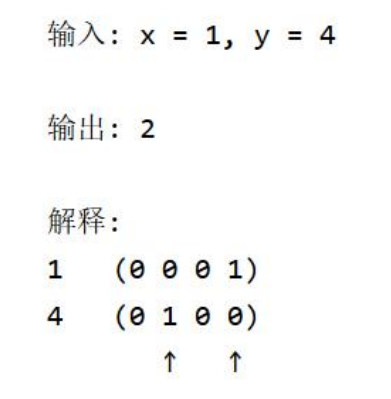均值哈希算法

步骤：

1. 缩放：图片缩放为8*8，保留结构，除去细节。
2. 灰度化：转换为灰度图。
3. 求平均值：计算灰度图所有像素的平均值。
4. 比较：像素值大于平均值记作1，相反记作0，总共64位。
5. 生成hash：将上述步骤生成的1和0按顺序组合起来既是图片的指纹（hash）。
6. 对比指纹：将两幅图的指纹对比，计算汉明距离，即两个64位的hash值有多少位是不一样的，不 相同位数越少，图片越相似。

差值哈希算法

差值哈希算法相较于均值哈希算法，前期和后期基本相同，只有中间比较hash有变化。

步骤：

1. 缩放：图片缩放为8*9，保留结构，除去细节。
2. 灰度化：转换为灰度图。
3. 求平均值：计算灰度图所有像素的平均值。
4. 比较：像素值大于后一个像素值记作1，相反记作0。本行不与下一行对比，每行9个像素， 八个差值，有8行，总共64位
5. 生成hash：将上述步骤生成的1和0按顺序组合起来既是图片的指纹（hash）。
6. 对比指纹：将两幅图的指纹对比，计算汉明距离，即两个64位的hash值有多少位是不一样 的，不相同位数越少，图片越相似。

感知哈希算法

均值哈希算法过于严格，不够精确，更适合搜索缩略图，为了获得更精确的结果可以选择感知哈希 算法，它采用的是DCT（离散余弦变换）来降低频率的方法。

步骤：

1. 缩小图片：32 * 32是一个较好的大小，这样方便DCT计算
2. 转化为灰度图：把缩放后的图片转化为灰度图。
3. 计算DCT:DCT把图片分离成分率的集合
4. 缩小DCT：DCT计算后的矩阵是32 * 32，保留左上角的8 * 8，这些代表图片的最低频率。
5. 计算平均值：计算缩小DCT后的所有像素点的平均值。
6. 进一步减小DCT：大于平均值记录为1，反之记录为0.
7. 得到信息指纹：组合64个信息位，顺序随意保持一致性。
8. 最后比对两张图片的指纹，获得汉明距离即可。

代码实现：均值哈希算法和差值哈希算法

import cv2
import numpy as np

#均值哈希算法
def aHash(img):
#缩放为8*8
img=cv2.resize(img,(8,8),interpolation=cv2.INTER_CUBIC)
#转换为灰度图
gray=cv2.cvtColor(img,cv2.COLOR_BGR2GRAY)
#s为像素和初值为0，hash_str为hash值初值为''
s=0
hash_str=''
#遍历累加求像素和
for i in range(8):
for j in range(8):
s=s+gray[i,j]
#求平均灰度
avg=s/64
#灰度大于平均值为1相反为0生成图片的hash值
for i in range(8):
for j in range(8):
if  gray[i,j]>avg:
hash_str=hash_str+'1'
else:
hash_str=hash_str+'0'
return hash_str

#差值感知算法
def dHash(img):
#缩放8*9
img=cv2.resize(img,(9,8),interpolation=cv2.INTER_CUBIC)
#转换灰度图
gray=cv2.cvtColor(img,cv2.COLOR_BGR2GRAY)
hash_str=''
#每行前一个像素大于后一个像素为1，相反为0，生成哈希
for i in range(8):
for j in range(8):
if   gray[i,j]>gray[i,j+1]:
hash_str=hash_str+'1'
else:
hash_str=hash_str+'0'
return hash_str

#Hash值对比
def cmpHash(hash1,hash2):
n=0
#hash长度不同则返回-1代表传参出错
if len(hash1)!=len(hash2):
return -1
#遍历判断
for i in range(len(hash1)):
#不相等则n计数+1，n最终为相似度
if hash1[i]!=hash2[i]:
n=n+1
return n

hash1= aHash(img1)
hash2= aHash(img2)
print(hash1)
print(hash2)
n=cmpHash(hash1,hash2)
print('均值哈希算法相似度：',n)

hash1= dHash(img1)
hash2= dHash(img2)
print(hash1)
print(hash2)
n=cmpHash(hash1,hash2)
print('差值哈希算法相似度：',n)

运行结果：

输入：输出：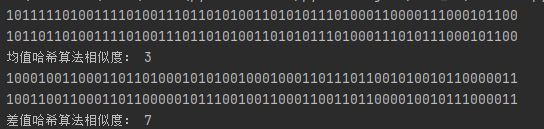图像相似度比较哈希算法

三种算法的比较：
• aHash：均值哈希。速度比较快，但是常常不太精确。
• pHash：感知哈希。精确度较高，但是速度方面较差一些。
• dHash：差值哈希。精确度较高，且速度也非常快。
• 均值哈希本质上是对颜色的比较；
• 感知哈希由于做了 DCT 操作，本质上是对频率的比较；
• 差值哈希本质上是基于渐变的感知哈希算法。

展开全文q923714892 2021-06-02 13:52:05
• 数据结构与算法实例(哈希表实现) 数据结构 算法 c语言

数据结构算法哈希表） 哈希函数：在记录的关键字与记录的存储地址之间建立的一 种对应关系叫哈希函数。 哈希函数是一种映象，是从关键字空间到存储地址空间的一 种映象。可写成：addressi=H(keyi) ，其中i是表中...

数据结构与算法（哈希表）

哈希函数：在记录的关键字与记录的存储地址之间建立的一 种对应关系叫哈希函数。
哈希表：应用哈希函数，由记录的关键字确定记录在表中的 地址，并将记录放入此地址，这样构成的表叫哈希
★哈希表的特点就是数据与其在表中的位置存在相关性，也就是有关系的，通过数据应该可以计算其位置，哈希表最大的特点是是可以快速实现查找，插入和删除。因为它独有的特点，Hash表经常用来解决大数据问题
1.哈希表的基本思想
数组的最大的特点是：寻址容易，插入和删除困难；而链表正好相反，寻址困难，而插入和删除操作容易。那么如果能够结合两者的优点。做出一种寻址，插入和删除操作同样快速的数据结构，这就是哈希表。哈希表是这样一个集查找，插入和删除操作于一身的数据结构

2.哈希表结构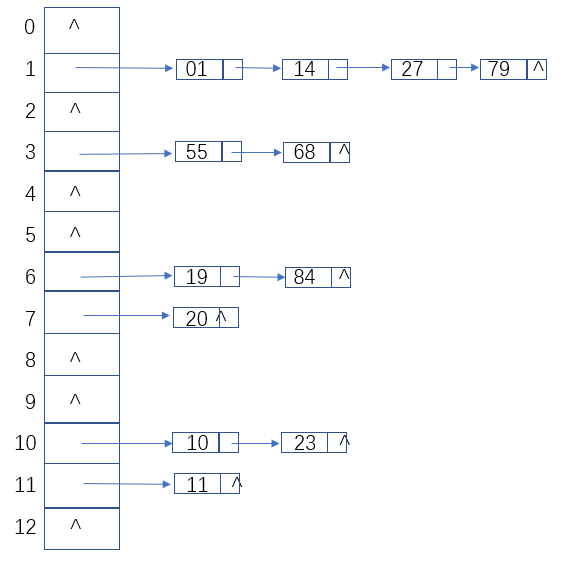3.基本操作

//创建结构体存储键值对
struct element {
int key;
int value;
};
//创建结构体作为一级结构
struct table;
//初始化哈希表存储空间，返回指向该空间的结构体指针变量
struct table *table_init();
void table_free(struct table *t);

void table_clear(struct table *t);
int table_isempty(struct table *t);
int table_count(struct table *t);
//将新的键值对存储到哈希表存储结构中
void table_put(struct table *t, int key, int value);
// void table_put(struct table *t, struct element e);
//根据键值将键值对从哈希表存储结构中移除
struct element table_remove(struct table *t, int key);

/* 如果找到了返回key的元素，如果没找到返回{-1,-1}*/
struct element table_get(struct table *t, int key);

4.存储结构
结构①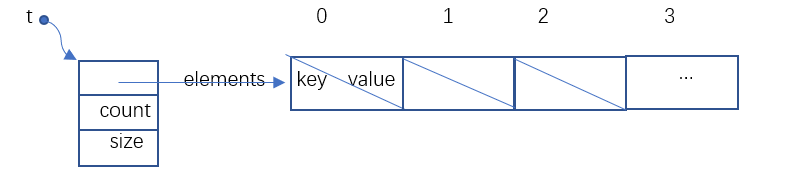核心思想:使用结构体数组存储键值对作为哈希表的存储结构核心

结构②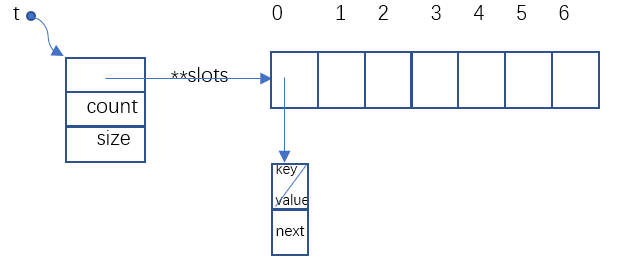核心思想:将链表连接到数组中，数组变量作为各链表的索引达到方便操作数据的目的

5.代码实现
table.h

#ifndef __TABLE_H__
#define __TABLE_H__
//定义结构体存储键值对
struct element {
int key;
int value;
};

struct table;

struct table *table_init();
void table_free(struct table *t);

void table_clear(struct table *t);
int table_isempty(struct table *t);
int table_count(struct table *t);

void table_put(struct table *t, int key, int value);

struct element table_remove(struct table *t, int key);

struct element table_get(struct table *t, int key);

#endif

main.c

//将数据元素按照键值关系存储到哈希表存储结构中
#include <stdio.h>
#include <stdlib.h>

#include "table.h"

int main(int argc, char *argv[])
{
//定义结构体指针变量t指向table结构体类型的数据
struct table *t = NULL;
int i;
//定义element结构体类型变量e
struct element e;
//t指向已开辟的内存空间
t = table_init();

table_put(t,0,55);
table_put(t, 1, 100);
table_put(t, 2, 200);
table_put(t, 3, 300);
table_put(t, 5, 500);

//键相同会产生"冲突",键不变，值覆盖
table_put(t, 3, 333);
//循环遍历，将键值取出
for (i=0; i<10; i++) {
e = table_get(t, i);
printf("the element is <%d, %d>\n", e.key, e.value);
}

table_free(t);

system("PAUSE");
return 0;
}

table.c
结构⑴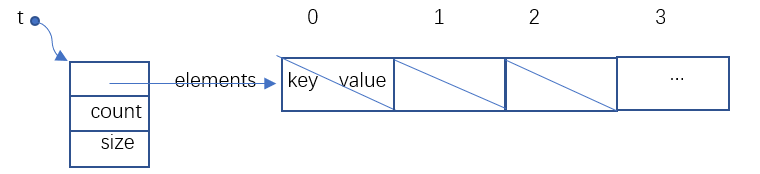#include "table.h"
#include <stdlib.h>
#include <assert.h>
#include <string.h>
//定义数组的初始长度
#define TABLE_INIT_SIZE 1
//定义数组每次扩容增加的长度
#define TABLE_INCR_SIZE 1
//定义table结构体类型作为一级结构体，指针变量elements指向在.h文件中定义的element结构体类型数据元素
//count记录结构体数组中数据元素的个数，size记录结构体数组的长度
struct table {
struct element *elements;
int count;
int size;
};
//创建以结构体数组为核心的哈希表存储结构
struct table *table_init()
{
struct table *t = NULL;

t = (struct table *)malloc(sizeof(struct table));
if (t == NULL) return NULL;

assert(t != NULL);
t->elements = NULL;
t->size = 0;
t->count = 0;

t->elements = (struct element *)malloc(sizeof(struct element) * TABLE_INIT_SIZE);
if (t->elements == NULL) {
free(t);
return NULL;
}

assert(t->elements != NULL);

memset(t->elements, 0, sizeof(struct element) * TABLE_INIT_SIZE);
t->size = TABLE_INIT_SIZE;

return t;
}
//通过table_free()释放内存空间
void table_free(struct table *t)
{
assert(t != NULL);
assert(t->elements != NULL);

free(t->elements);
free(t);

return;
}
//清空哈希表存储结构中的数据元素
void table_clear(struct table *t)
{
assert(t != NULL);
assert(t->elements != NULL);

t->count = 0;

return;
}
//查看哈希表存储结构中是否为空
int table_isempty(struct table *t)
{
assert(t != NULL);
assert(t->elements != NULL);

return (t->count == 0);
}
//计算哈希表存储结构中数据元素的个数
int table_count(struct table *t)
{
assert(t != NULL);
assert(t->elements != NULL);

return t->count;
}
//将键值对push到哈希表存储结构中
void table_put(struct table *t, int key, int value)
{
//如果已存储的数据元素等于已定义的数组的长度，进行扩容操作
if (t->count == t->size) {
t->elements = (struct element *)realloc(t->elements, sizeof(struct element) * (t->size + TABLE_INCR_SIZE));
if (t->elements == NULL) {
perror("struct table_put realloc error");
exit(1);
}

t->size += TABLE_INCR_SIZE;
}

struct element e = {key, value};
int i=0;
//如果产生"冲突",键不变，值覆盖
for (; i<t->count; i++) {
if (t->elements[i].key == e.key) {
t->elements[i].value = e.value;
return;
}
}

t->elements[i] = e; // t->count
t->count++;
return;
}

// void table_put(struct table *t, struct element e);
//将制定键的键值对移除
struct element table_remove(struct table *t, int key)
{
struct element e = {-1, -1};
int i=0;
//定义pos标识键相同与制定键相同的键值对所在结构体中的位置
int pos = -1;

for (; i<t->count; i++) {
e=t->elements[key];
if (t->elements[i].key == key) {
pos = i;
break;
}
}
//后面的数据元素往前移位
if (pos != -1) {
e = t->elements[pos];
for (; pos < t->count-1; pos++) {
t->elements[pos] = t->elements[pos+1];
}
t->count--;
}

return e;
}

struct element table_get(struct table *t, int key)
{
int i;
struct element e = {-1, -1}; /*未找到返回的值*/

for (i=0;i<t->count; i++) {
if (t->elements[i].key == key) return t->elements[i];
}

return e;
}

结构⑵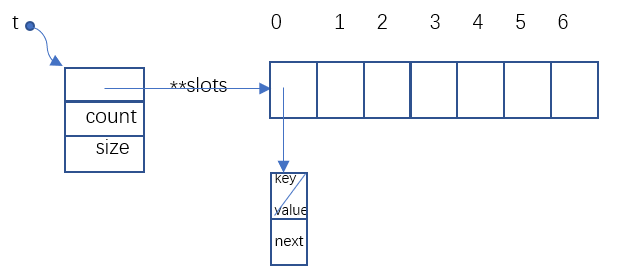#include "table.h"

#include <stdlib.h>
#include <assert.h>
#include <string.h>
//定义作为索引的数组的长度
#define TABLE_SLOTS_INIT_SIZE 10
//定义table_node的结构体类型作为链表的数据节点，e为element结构体类型的数据元素（element结构体在.h文件中已定义）
//next指向下一个table_node数据类型的数据
struct table_node {
struct element e;
struct table_node *next;
};
//table结构体类型作为一级结构，数据节点地址存储在数组中，数组的首地址存放在slots指针变量中
struct table {
struct table_node **slots;
int count;
int size;
};

struct table *table_init()
{
struct table *t = NULL;

t = (struct table *)malloc(sizeof(struct table));
if (t == NULL) return NULL;

assert(t != NULL);
t->slots = NULL;
t->count = 0;
t->size = 0;

t->slots = (struct table_node **)malloc(sizeof(struct table_node *) * TABLE_SLOTS_INIT_SIZE);

if (t->slots == NULL) {
free(t);
return NULL;
}

assert(t->slots != NULL);

memset(t->slots, 0, sizeof(struct table_node *) * TABLE_SLOTS_INIT_SIZE);

t->size = TABLE_SLOTS_INIT_SIZE;

return t;
}

void table_free(struct table *t)
{
struct table_node *node = NULL;
int i;

for (i=0; i<t->count; i++) {
while (t->slots[i] != NULL) {
node = t->slots[i];
t->slots[i] = node->next;
free(node);
}

assert(t->slots[i] == NULL);
}

free(t->slots);
free(t);

return;
}

void table_clear(struct table *t)
{
struct table_node *node = NULL;
int i;

assert(t != NULL);
assert(t->slots != NULL);

for (i = 0; i < t->count; i++) {
while (t->slots[i] != NULL) {
node = t->slots[i];
t->slots[i] = node->next;
free(node);
}

assert(t->slots[i] == NULL);
}

t->count = 0;

return;
}

int table_isempty(struct table *t)
{
assert(t != NULL);
assert(t->slots != NULL);

return (t->count == 0);
}

int table_count(struct table *t)
{
assert(t != NULL);
assert(t->slots != NULL);

return (t->count);
}

void table_put(struct table *t, int key, int value)
{
struct table_node *p = NULL;
struct table_node *node = NULL;

p = t->slots[key % t->size];
while (p != NULL) {
if (p->e.key == key) {
p->e.value = value;
return;
}
p = p->next;
}

node = (struct table_node *)malloc(sizeof(struct table_node));
if (node == NULL) {
perror("table_put malloc error");
exit(1);
}

assert(node != NULL);
node->e.key = key;
node->e.value = value;

/* 把链上结点挂在node结点之后，注意，链上没有结点也没关系*/
node->next = t->slots[key % t->size];
t->slots[key % t->size] = node;
t->count++;

return;
}

// void table_put(struct table *t, struct element e);
struct element table_remove(struct table *t, int key)
{
struct table_node *p = NULL;
struct table_node *node = NULL;
struct element e = {-1, -1};

p = t->slots[key % t->size];

if (p->e.key == key) {
node = p;
t->slots[key % t->size] = node->next;
e = node->e;
free(node);
t->count--;
return e;
}

while (p != NULL && p->next != NULL) {
if (p->next->e.key == key) {
node = p->next;
p->next = node->next;
e = node->e;
t->count--;
free(node);
return e;
}
p = p->next;
}

return e;
}

/* 如果找到了返回key的元素，如果没找到返回{-1,-1}*/
struct element table_get(struct table *t, int key)
{
struct table_node *p = NULL;
struct element e = {-1, -1};

p = t->slots[key % t->size];
while (p != NULL) {
if (p->e.key == key) {
e = p->e;
break;
}
p = p->next;
}

return e;
}

6.编译结果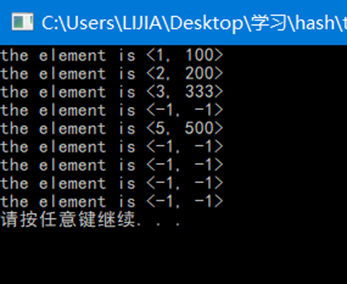展开全文lucklycoder 2021-01-25 16:18:14
• 数据结构与算法-哈希算法-Hash函数&装载因子&动态扩容 数据结构 算法 java

Hash函数的确定 ...其次， Hash函数生成的值要尽可能随机并且均匀分布，这样才能避免或者最小化散列冲突，而且即使出现冲突，散列到每个槽中的数据也会比较平均，不会导致某些槽中的数据过多，而另一

Hash函数的确定

通过前面学习到， Hash表的查询效率并不是 O(1)，它与 Hash函数、散列冲突等因素有关。如果 Hash函数确定得不好，可能导致散列冲突概率升高，查询效率下降。那么，该如何设计 Hash函数呢？

首先， Hash函数一般设计得不要过于复杂，过于复杂的 Hash函数会导致计算时间过多，从而影响散列表的性能；

其次， Hash函数生成的值要尽可能随机并且均匀分布，这样才能避免或者最小化散列冲突，而且即使出现冲突，散列到每个槽中的数据也会比较平均，不会导致某些槽中的数据过多，而另一部分槽中的数据过少的情况。

传统的 Hash函数的设计方法有直接寻址法、平方取中法、折叠法、随机数法等，也可以根据实际情况自己设计 Hash函数。

装载因子的确定

为了定量的表示 Hash表中空位的多少，定义装载因子

Hash表的装载因子 = 填入表中的元素个数 / Hash表的长度

由公式可知，装载因子越大，说明 Hash表中的元素越多，空闲位置越少，散列冲突的概率越大，散列表的性能就会下降。

对于没有频繁插入和删除的静态数据结合来说，可以根据数据的特点和分布情况设计出符合这些数据的 Hash函数，从而减少了散列冲突。

但是大部分情况下是动态数据，数据集合是频繁变动的，我们无法事先知道数据的个数，因此也无法事先申请一个足够大的 Hash表。随着数据加入，填入表中的元素个数增多，装载因子增大，当装载因子达到一定程度时，散列冲突便不可接受，因此我们无法根据数据的特征和分布情况设计出符合这些数据的 Hash函数，而是需要动态扩容，重新申请一个更大的 Hash表，将数据重新存储到新的 Hash表中。

如下图中的散列表，当装载因子达到0.8时进行扩容，装载因子变为0.4，原来的数据就会存储在新的 Hash表中。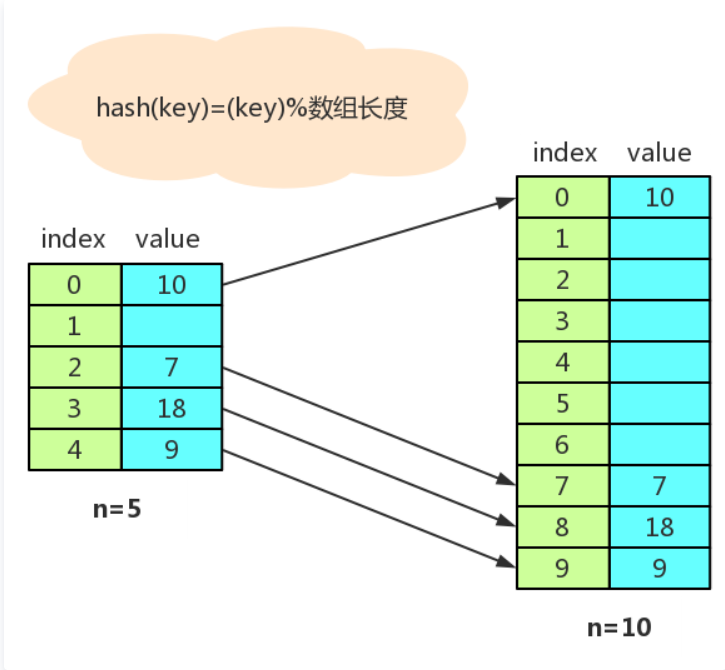当数据插入到 Hash表时，如果装载因子还未达到临界值，此时还不需要扩容，插入的数据非常快，但如果装载因子达到了临界值，这是就需要先进行扩容，然后再插入数据，这个时候就会变得很慢。

当数据需要从 Hash表中删除时，如果 Hash表已经经历过扩容，随着数据的删除，空闲空间会越来越多。当程序对内存空间非常敏感时，可以设置当装载因子小于某个临界值时，启动动态缩容，让内容空间得到充分利用；当程序对内存空间不太敏感时，就不需要进行动态缩容处理。

动态扩容策略

为了减少动态扩容耗时，我们可以将扩容的操作穿插在插入操作过程中。具体如下图所示：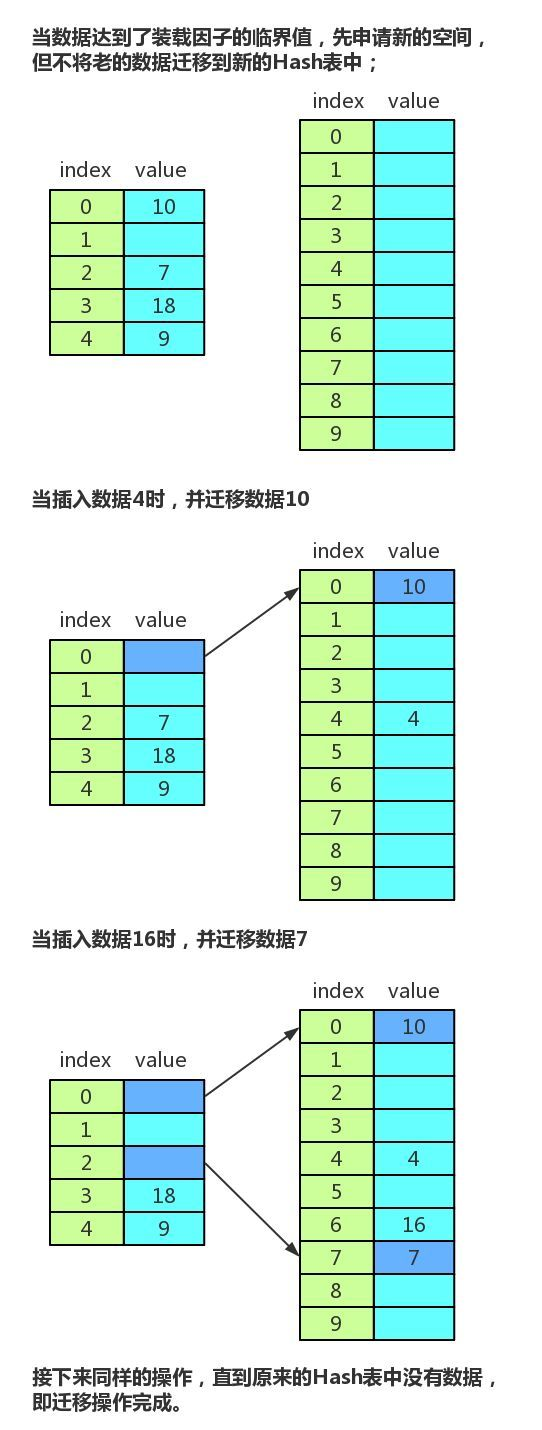这样每次插入时迁移一个数据，没有集中一次性迁移数据那样耗时，不会形成明显的阻塞。

由于迁移过程中，有新旧两个 Hash表，查找数据时，先在新的 Hash表中进行查找，如果没有，再去旧的 Hash表中进行查找。

展开全文younow22 2021-01-04 14:53:23
• 查找-顺序+折半+索引+哈希【数据结构与算法】 数据结构 二分查找 哈希算法 顺序查找 折半查找

weixin_44972997 2021-02-17 15:34:19
• Java数据结构与算法——原地哈希算法 算法 数据结构 java leetcode

qq_43409401 2021-04-17 10:27:49
• 数据结构哈希查找 数据结构 哈希表

weixin_44581918 2021-01-30 22:36:04
• 数据结构和算法_哈希算法_5 算法

qq_42642159 2021-05-27 16:02:50
• 哈希算法介绍 哈希算法

weixin_44109140 2021-12-12 16:13:52
• 数据结构与算法——哈希表及哈希扩容（Java实现） 数据结构 算法 java

nswdiphone6 2021-02-25 21:53:35
• 数据结构和算法-哈希 数据结构 算法

zhuzi121121 2020-12-29 10:41:55
• 【数据结构与算法】算法的特性以及查找算法（顺序，二分，哈希，块） 算法 数据结构 c语言 二分查找 哈希算法

weixin_54057383 2021-08-19 19:24:02
• 数据结构与算法之美 - 21 | 哈希算法（上）：如何防止数据库中的用户信息被脱库？ 算法

aha_jasper 2021-01-06 17:09:01
• qq_20853741 2020-12-30 17:40:00
• qq_16570607 2021-07-11 20:38:07
• 哈希算法 算法 java 分布式

weixin_47251999 2021-01-24 18:46:54
• kalision 2021-04-20 13:27:53
• C++数据结构与算法——哈希表实现（链式法） 数据结构 算法 c++ 散列表

qq_39420306 2021-11-24 13:32:13
• 算法与数据结构（五）哈希表 算法 数据结构 hashtable 哈希表

u013647453 2021-05-07 23:36:14
• 和你聊聊哈希算法 算法 python 数据结构 java 数据库

qq_33254766 2021-06-15 14:07:14
• JavaScript数据结构与算法 - 哈希表详解 算法 数据结构 javascript

weixin_45663697 2021-05-31 10:44:30
• Java哈希表数据结构 java hashmap 数据结构 哈希表 map

weixin_43721056 2021-03-14 14:48:54
• 数据结构定义和算法--哈希算法（下） 算法

ruzewei 2021-01-18 20:44:10
• 数据结构之哈希表 数据结构 散列表 哈希算法

jane_xing 2021-11-10 09:33:24
• 数据结构与算法之查找算法——哈希表（又称散列表） 数据结构 算法 散列表 哈希算法

qq_44631615 2021-10-31 11:24:14
• 【数据结构】可以逃课其它字符串算法的字符串哈希算法 算法 数据结构 c语言

qq_49688477 2021-09-17 14:05:21
• weixin_34972620 2021-03-17 18:42:20
• 【数据结构】【哈希】哈希表的概念和算法实现 数据结构 算法 哈希 c++

qq_45520647 2021-08-31 22:08:59
• 数据结构与算法之美 - 22 | 哈希算法（下）：哈希算法在分布式系统中有哪些应用？ 数据结构 算法

aha_jasper 2021-01-06 17:28:56
• 数据结构 哈希查找 数据结构

m0_46326495 2021-09-18 00:07:36...

哈希算法数据结构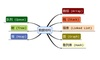数据结构 订阅